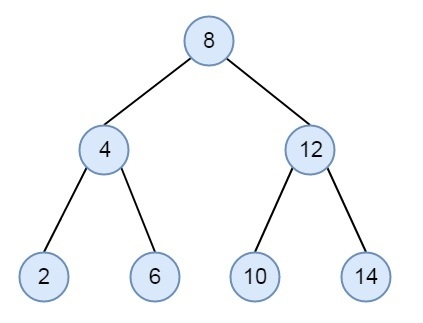# Floor and Ceil from a BST in C++

Here we will see, how to find the Floor and Ceiling value from BST. For example, if we want to make a memory management system, where free nodes are arranged in BST. Find best fit for the input request. Suppose we are moving down the tree with smallest data larger than the key value, then there are three possible cases.

• Root is the key. Then root value is the ceiling value
• If root data < key, then the ceiling value will not be at the left subtree, then proceed to right subtree, and reduce the problem domain
• If root data > key, then the ceiling value may be at right subtree, we may find a node with larger data than key in left subtree, if no such element is present, then root is the ceiling value.

Suppose the tree is like this −Ceiling of 0, 1, 2 is 2, ceiling of 3, 4 is 4 and so on

Here we will see the ceiling function only, if we modify this little bit, we can get the floor values.

## Example

Live Demo

#include <iostream>
using namespace std;
class node {
public:
int key;
node* left;
node* right;
};
node* getNode(int key) {
node* newNode = new node();
newNode->key = key;
newNode->left = NULL;
newNode->right = NULL;
return newNode;
}
int ceiling(node* root, int num) {
if (root == NULL)
return -1;
if (root->key == num)
return root->key;
if (root->key < num)
return ceiling(root->right, num);
int ceil = ceiling(root->left, num);
return (ceil >= num) ? ceil : root->key;
}
int main() {
node* root = getNode(8);
root->left = getNode(4);
root->right = getNode(12);
root->left->left = getNode(2);
root->left->right = getNode(6);
root->right->left = getNode(10);
root->right->right = getNode(14);
for (int i = 0; i < 16; i++)
cout << i << "\tCeiling: " << ceiling(root, i) << endl;
}

## Output

0 Ceiling: 2
1 Ceiling: 2
2 Ceiling: 2
3 Ceiling: 4
4 Ceiling: 4
5 Ceiling: 6
6 Ceiling: 6
7 Ceiling: 8
8 Ceiling: 8
9 Ceiling: 10
10 Ceiling: 10
11 Ceiling: 12
12 Ceiling: 12
13 Ceiling: 14
14 Ceiling: 14
15 Ceiling: -1